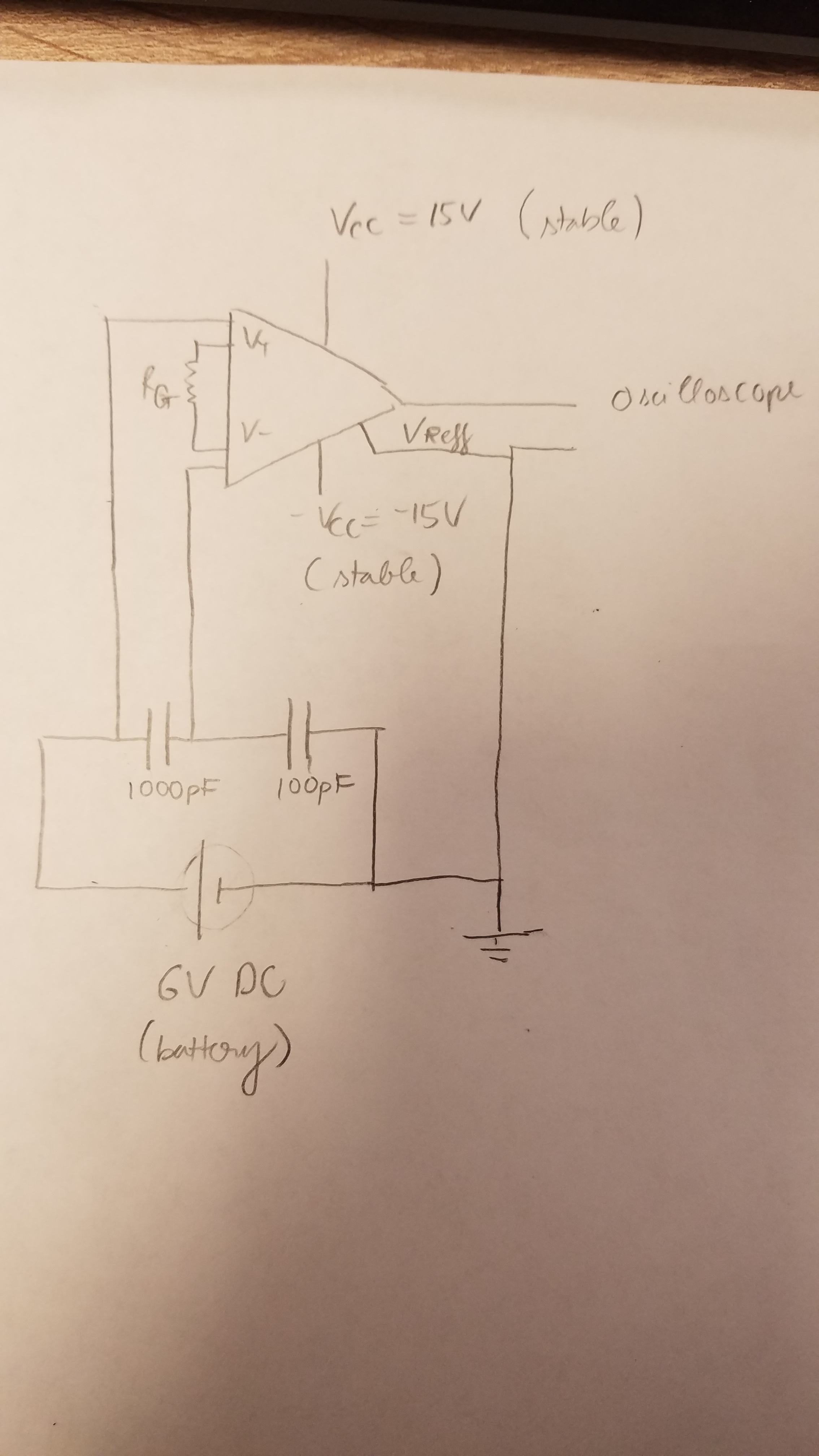If you have a related question, please click the "Ask a related question" button in the top right corner. The newly created question will be automatically linked to this question.

# INA116: Capacitance Measurement Issue

Part Number: INA116

Dear TI Technical Support,

I am currently working on a measuring circuit that measure the capacitance in a DC circuit. I have posted my Schematics  in this post.

The output readout at the oscilloscope screen is not as we expected. As the voltage across the two capacitors is 6V, we expected to see the voltage across the larger capacitor to be 1.1V with RG = 50kOhm, and 0.55 with RG = infinity (not connected).

1. However, while the VCC and -VCC are held stable at 15V and -15V, there are occasions that the oscilloscope still read a few volts even when the battery to the two capacitors are disconnected.

2. The output of the oscilloscope seems to be influenced by electromagnetic interference. When  I curbed my hand and periodically move my hand closer to or away from the two Vin+ and Vin- wires, there was oscillation at the readout.

3. When i switched the order of the two capacitors but still measuring across the larger capacitor, the voltage readout of the oscilloscope was not the same.

4. Sometimes, I see the voltage readout is reversed, sometimes it was upright.

I did connect guard- to guard- and guard+ to guard+ pins. I also connected two pairs of capacitor and resistor to ground like the figure 3 page 8 of the datasheet, but the behavior persists. I also made sure that the common-mode voltage of the measured capacitor is small (it was about 250mV).

I understand that some of these issues are very simple to fix, but this is my first time working with op amps in general. So I really appreciate your support.

Sincerely,

Khoi Ly• Hi Khoi,

First off, you definitely need to include the resistors to ground at the inputs of the INA116. Without these, despite the fact that the bias current for this device is very low, it will still result in abnormal behavior as the current charges and discharges the capacitors on the input. The resistors should be tied to the same ground as your power supplies. You may also want to include resistors in series with your battery (like figure 3) to limit the current spike as you apply voltage to the capacitors.

It looks to me like you are tying the negative terminal of the battery to the same ground potential as your power supplies, is this correct?
• Hi zak,

When I place 2 resistors from the large capacitor (see text below) to ground as you said, it keeps discharging to ground and the in amp measurement gradually decays to zero.

But without these resistors, It seems like I have to drain the two capacitors completely (by shorting them out) after each measurement otherwise the readout is not correct.

let's say in theory I apply a voltage of 250V to the two capacitors in series (10000pF and 100pF). I should expect, at steady state, to receive voltage across the large capacitor to be 2.47V (capacitance in series equations. So with inamp's gain of 2 (resistor gain =50kohm), I expect to see the output voltage to be 5V.

However, at initial interval time, the output is always starting higher than 5V (around 8V), then gradually decay and stablize around 5V. How do I get 5V immediately? The issue was the same for different gain/Vsupply/and Capacitance values.
• Hi Khoi,

The input resistors are recommended because right now your bias current doesn't have a dc path to ground. Since the bias current of the INA116 is so low the issue would likely manifest as a drifting output voltage as the bias currents charge/discharge the caps, which is likely what you were seeing when the output was drifting without an input voltage. Since the bias current is so low, there is room to make the resistors quite large without introducing too much error. This would help to maintain the voltage across the capacitor while you take the reading.

Can you describe what exactly you are looking for from this circuit? What range of capacitance do you need to measure? Where is the output of the INA intended to go? How long do you need the voltage reading to remain valid? You may be able to do something like follow the INA with a peak detector circuit to hold the voltage for longer if necessary.

What do you mean when you say "gradually decays"? Is this over the course of milliseconds, seconds? If you could provide oscilloscope plots including your configuration this would also be helpful.